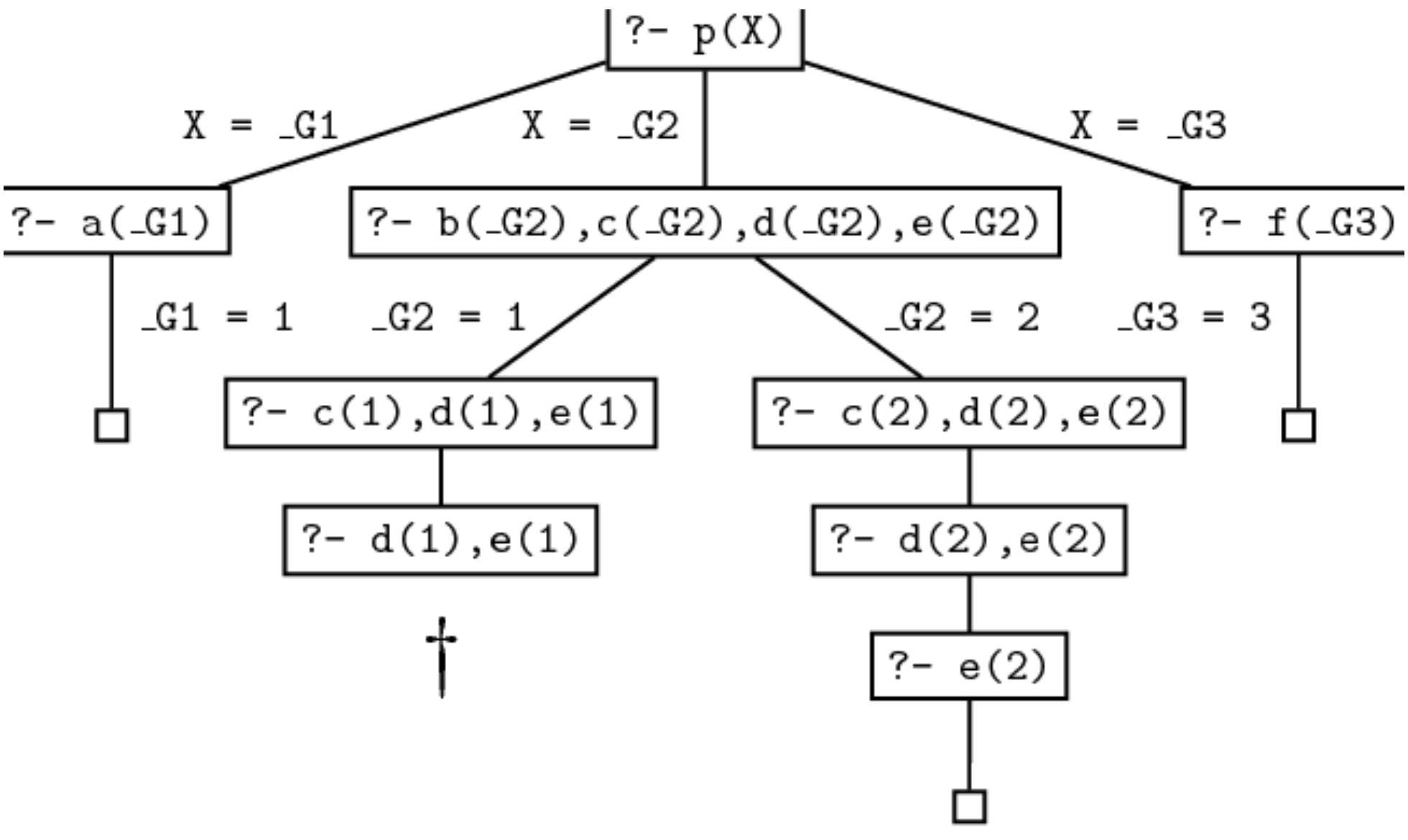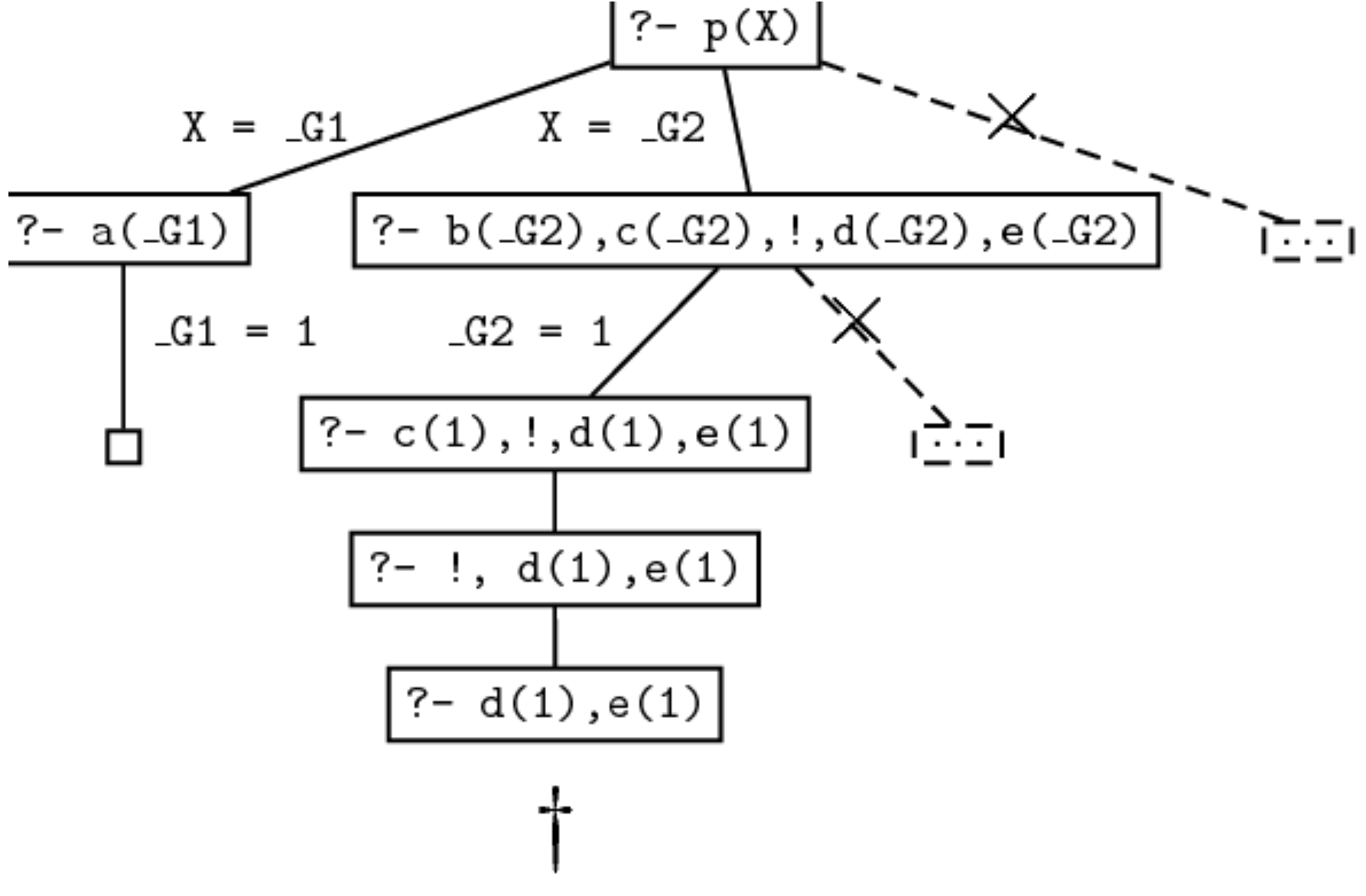# Prolog 学习 Ch6

## Cut and Negation

Posted by R1NG on October 31, 2021 Viewed Times

# Prolog 入门: 剪枝与回溯

## 1. 剪枝谓词 Cut

Prolog 的证明搜索机制高度依赖自动化的回溯算法, 但基于预置规则的回溯行为有时可能会导致遍历了完全没有必要遍历的内容, 降低程序的运行效率.

Cut 的占位符为 !, 它是一个可被直接写入子句中的原子谓词:

1
axton(X) :- rhythm_gamer(X), proficient_developer(X), !, android_user(X), windows_user(X).


1. 在证明搜索过程中若它被视为目标, 则它为一个 永远被满足的目标.
2. 考虑某个子句 $\alpha$ 包含了剪枝谓词 !. 则在某次为了尝试满足目标 $G$ 的证明搜索尝试联合该子句时, 剪枝谓词会 直接将自目标 $G$ 和包含子句的规则左侧联合之前所执行的所有选择, 包括对这个特定子句 $\alpha$ 的选择作为查询的结果提交, 即使联合失败程序也不会再尝试新的规则或变量.

1
2
3
4
5
6
7
p(X) :- a(X).
p(X) :- b(X), c(X), d(X), e(X).
p(X) :- f(X).

a(1). b(1). c(1).
d(2). e(2). f(3).
b(2). c(2).


1
2
3
4
5
6
?- p(X).
X = 1 ;
X = 2 ;
X = 3.

false1
p(X) :- b(X), c(X), !, d(X), e(X).


1
2
3
?- p(X).
X = 1 ;
false.


1. 在程序执行伊始 Prolog 尝试首先联合第一条规则, 因此我们得到目标 a(X). 通过检索知识库将 a 实例化为 $1$, 程序即可将 a(X) 和事实 a(1) 联合, 从而找到第一个可行解 X=1. 到此为止新程序的执行流程和原先没有任何差别.
2. 下面 Prolog 会尝试联合第二条规则, 而得到目标序列 b(X),c(X),!,d(X),e(X). 由于 Prolog 顺着知识库中事实排序尝试联合变量 X, 此次尝试还是会从 X=1 开始. 联合的结果使得我们满足了目标序列中的前两个子目标, 此时的目标序列变为 !,d(X),e(X).
3. 我们对第二条规则的修改在此处真正生效. 由于 ! 被视为一个自动被满足的目标, 因此程序会提交我们此前做出的选择 X=1 和我们当前对第二条规则的选择, 跳过 ! 并尝试联合下一个子目标 d(X).
4. 由于无法从知识库中找到满足目标的事实 d(1), 此次联合失败, 并且我们找不出任何能够满足该子目标的其他方法. 由于 Prolog 在处理内置谓词 ! 时已经将我们在这一轮证明搜索中对事实和规则的选择提交, 程序不再被允许回溯并重新选择事实 b(2) 进行联合, 也更不会被允许回溯到原点选择第三条规则进行联合, 因此我们只能得到在第一轮联合中得到的唯一解 X=1.1
q:-  p1,...,pn,  !,  r1,...,rm


## 2. 对剪枝谓词的利用

1
2
max(X,Y,Y) :- X =< Y.
max(X,Y,X) :- X > Y.


1
2
max(X,Y,Y) :- X =< Y, !.
max(X,Y,X) :- X > Y.


1
2
max(X,Y,Y) :- X =< Y, !.
max(X,Y,X).


1
?- max(114, 514, 114).


1
2
max(X,Y,Z) :- X =< Y, !, Y = Z.
max(X,Y,X).


## 3. 回溯谓词

1
enjoys(vincent, X) :- burger(X).


Prolog 内置的另一个谓词 fail/0 (回溯谓词) 就可以实现这一点. 和 ! 类似, fail 可被视为一个始终 无法得到满足的目标, 因此在任何一轮证明搜索时如果 fail 作为子目标之一, 那么证明搜索就无法继续下去而被迫执行回溯, 也就是说 fail强制程序在当前节点回溯 的作用. 当回溯谓词和剪枝谓词结合时, 我们就可以一并利用它们各自的性质完成对 “特例” 的实现.

1
2
3
4
5
6
7
8
9
10
11
enjoys(vincent,X) :- big_kahuna_burger(X),!,fail.
enjoys(vincent,X) :- burger(X).

burger(X) :- big_mac(X).
burger(X) :- big_kahuna_burger(X).
burger(X) :- whopper(X).

big_mac(a).
big_kahuna_burger(b).
big_mac(c).
whopper(d).


1
2
neg(Goal) :- Goal, !, fail.
neg(Goal).


1
enjoys(vincent, X) :- burger(X), neg(big_kahuna_burger(X)).


1
enjoys(vincent, X) :- burger(X), \+ big_kahuna_burger(X).


1
2
3
4
5
6
7
8
9
10
enjoys(vincent, X) :- burger(X), \+ big_kahuna_burger(X).

burger(X) :- big_mac(X).
burger(X) :- big_kahuna_burger(X).
burger(X) :- whopper(X).

big_mac(a).
big_kahuna_burger(b).
big_mac(c).
whopper(d).